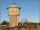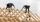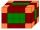Balls

Ping pong balls have a diameter of approximately 4.6 cm. They are sold in boxes of 4 pieces: each box has the shape of a cuboid with a square base. The balls touch the walls of the box.

Calculate what portion of the internal volume of the box is filled with balls.

Result

x =  52.36 %

Solution:Leave us a comment of example and its solution (i.e. if it is still somewhat unclear...):Be the first to comment!Next similar examples:

1. PersonsPersons surveyed:100 with result: Volleyball=15% Baseball=9% Sepak Takraw=8% Pingpong=8% Basketball=60% Find the average how many like Basketball and Volleyball. Please show your solution.
2. GasholderThe gasholder has spherical shape with a diameter 20 m. How many m3 can hold in?
3. Water reservoirThe reservoir shape of a cylinder having a diameter 8 m and a height 2.8 m. The device indicates that the reservoir contains 800 hectoliters of water. Calculate how much % of volume is used.
4. Percentage 1052 shorts and missed 13. Calculate percentage
5. Volume increaseHow many percent will increase in the pool 50 m, width 15m if the level rises from 1m to 150cm?
6. PercentsHow many percents is 900 greater than the number 750?
7. Profit gainIf 5% more is gained by selling an article for Rs. 350 than by selling it for Rs. 340, the cost of the article is:
8. Highway repairThe highway repair was planned for 15 days. However, it was reduced by 30%. How many days did the repair of the highway last?
9. The ballThe ball was discounted by 10 percent and then again by 30 percent. How many percent of the original price is now?
10. Theorem proveWe want to prove the sentence: If the natural number n is divisible by six, then n is divisible by three. From what assumption we started?Calculate how many percent will increase the length of an HTML document, if any ASCII character unnecessarily encoded as hexadecimal HTML entity composed of six characters (ampersand, grid #, x, two hex digits and the semicolon). Ie. space as: &#x20;52 is what percent of 93?From wooden block carpenter cut off a small cuboid block with half the edge length. How many percent of wood he cut off?How many % of the volume of the cube whose edge is 6 meters long is a volume of a sphere inscribed in that cube?Calculate the total volume of all cuboids for which the the size of the edges are in a ratio of 1:2:3, and one of the edges has a size 6 cm.Wjat height reach water level in the tray shaped a cuboid, if it is 420 liters of water and bottom dimensions are 120 cm and 70 cm.By how many percent increases the volume of cuboid if its every dimension increases by 30%?